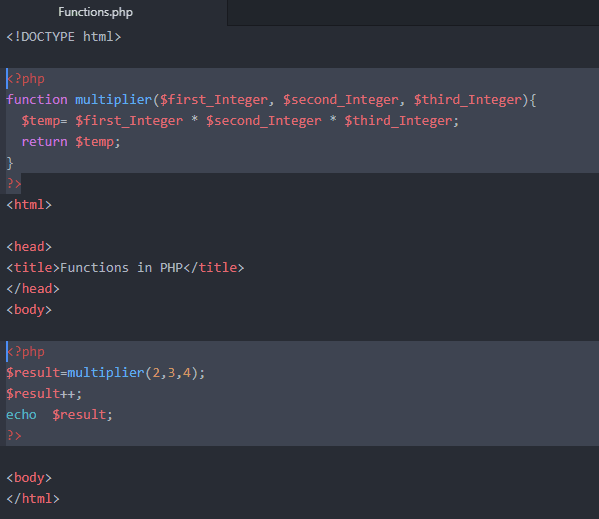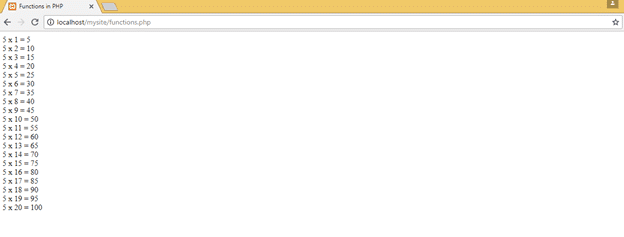## How to do PHP Tutorial on Functions in PHP

Functions in PHP – From our previous tutorials, we discussed some basic functions. For example, we used a count(); function to get the length of an array. It was a built-in function, there are plenty of others as well. So today, we will actually create some functions of our own and use them. But before we start you must understand the scope of a function. Every functioned defined in one page can only be accessible to that specific page only, you can also create a separate file of functions that you need in your web page and link this file to all the webpages.

##### Function without any Argument- Functions in PHP

A function can be defined using a very simple structure. First write the keyword “function” then after a space write the name of your function following with ‘()’ and finally for the boundary we write ‘{}’. Using this kind of syntax a simple function will be like

function print_Hello(){

echo “Hi there!!!”;

}

Now, once a function is defined we can call it within that file using its name with ‘()’ e.g. print_Hello();

Here is how it will look like in the codeIt is clear from the above example that you are free to define a function anywhere you want. But calling a function must be on where you want to place it. Since we wanted to output it in the body of the page, that’s why we called the function inside a body tag. Output of the code is

#####Functions with single Argument- Functions in PHP

There is a possibility to define a function that takes arguments and applies some functionality to them. For example, what if we write a function that’ll take a String and print it. Here

#####Function with more than one arguments– Functions in PHP

There could be more than 1 arguments in a function. We separate all the arguments with a comma just like this

function anyName(\$first, \$second, \$third,. . .)

Return Statement in a Function– Functions in PHP

Let’s say we need a function that takes 3 integers as arguments and multiply them. But we instead of printing the result want to use the resulting integer in some other calculations. This is possible using a return statement.It is clear from the example above that function returned an integer which we stored in a variable \$result. And we were also able to increment in this value before printing it. And as a result this value was printed.

#####Printing table by using a function- Functions in PHP

Since now we know how to use a function. It’s time to modify our previous code of printing a table and make it more generic. Here is a function that will take an integer and print its table.

<?php

function table_Of(\$int){

\$count=1;

for(\$i=0; \$i<20; \$i++){

echo “\$int x \$count = “.(\$int*\$count).”<br>”;

\$count++;

}

}

?>

And while calling it, we can add any integer we want. Example below shows running of the code with a value of 5.Out of this specific example is given below

#####Final Words– Functions in PHP

This is how we make our programs much more efficient. Can you go one step further? Here is the task you can perform to test your learning from this tutorial.

1. Make a function that takes 3 integer as input.
2. Print a table of first integer, starting from second integer and ending at third integer.
3. For example, if we call it this way table_Of(5,7,17); This output must be printed.